#### Making smaller information-feedback plans

The primary use of an I-map is to simplify the description of a plan. In Section 11.1.3, a plan was defined as a function on the history I-space,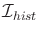. Suppose that an I-map,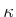, is introduced that maps fromto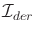. A feedback plan onis defined as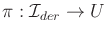. To execute a plan defined on, the derived I-state is computed at each stageby applyingto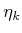to obtain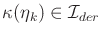. The action selected by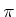is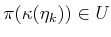.

To understand the effect of usinginstead ofas the domain of, consider the set of possible plans that can be represented over. Let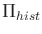and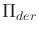be the sets of all plans overand, respectively. Any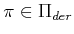can be converted into an equivalent plan,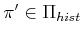, as follows: For each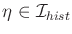, define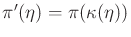.

It is not always possible, however, to construct a plan,, from some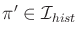. The problem is that there may exist some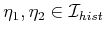for which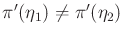and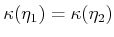. In words, this means that the plan inrequires that two histories cause different actions, but in the derived I-space the histories cannot be distinguished. For a plan in, both histories must yield the same action.

An I-maphas the potential to collapsedown to a smaller I-space by inducing a partition of. For each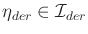, let the preimage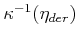be defined as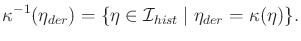(11.26)

This yields the set of history I-states that map to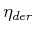. The induced partition can intuitively be considered as the resolution'' at which the history I-space is characterized. If the sets in (11.26) are large, then the I-space is substantially reduced. The goal is to selectto make the sets in the partition as large as possible; however, one must be careful to avoid collapsing the I-space so much that the problem can no longer be solved.

Example 11..11 (State Estimation)   In this example, the I-map is the classical approach that is conveniently taken in numerous applications. Suppose that a technique has been developed that uses the history I-stateto compute an estimate of the current state. In this case, the I-map is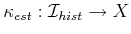. The derived I-space happens to bein this case! This means that a plan is specified as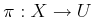, which is just a state-feedback plan.

Consider the partition ofthat is induced by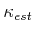. For each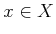, the set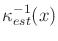, as defined in (11.26), is the set of all histories that lead to the same state estimate. A plan oncan no longer distinguish between various histories that led to the same state estimate. One implication is that the ability to encode the amount of uncertainty in the state estimate has been lost. For example, it might be wise to make the action depend on the covariance in the estimate of; however, this is not possible because decisions are based only on the estimate itself.Example 11..12 (Stage Indices)   Consider an I-map,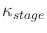, that returns only the current stage index. Thus,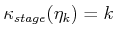. The derived I-space is the set of stages, which is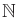. A feedback plan on the derived I-space is specified as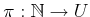. This is equivalent to specifying a plan as an action sequence,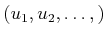, as in Section 2.3.2. Since the feedback is trivial, this is precisely the original case of planning without feedback, which is also refereed to as an open-loop plan.Steven M LaValle 2020-08-14